Latest Banking jobs   »   Reasoning Ability Quiz For RBI Assistant/...

# Reasoning Ability Quiz For RBI Assistant/ ESIC UDC Mains 2022- 21st April

Directions (1-5): Study the following information carefully and answer the questions accordingly.
Six people K, L, M, N, O, and P bought a car in six consecutive months starting from April in the same year but not necessarily in the same order. Each one bought the car of different colors i.e., Green, White, Black, Grey, Purple, and Brown but not necessarily in the same order.
Black car was not bought in June. P bought the car immediately before L. Two people bought the car between M and the Purple car. Two people bought the car between O and N. Neither K nor O bought the green car. L bought the Brown car after May. Purple car was bought immediately after O. N bought the White car in the month of 30 days but not in April.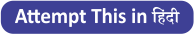Q1. Who bought the grey car?
(a) P
(b) M
(c) O
(d) K
(e) None of these

Q2. N bought the car in which of the following months?
(a) July
(b) June
(c) September
(d) August
(e) None of these

Q3. Car of which color is bought in April?
(a) White
(b) Brown
(c) Black
(d) Green
(e) None of these

Q4. How many people bought the car after K?
(a) Two
(b) Three
(c) One
(d) Four
(e) None of these

Q5. Find the correct combination?
(a) K – Grey
(b) L – July
(c) P – Purple
(d) O – April
(e) None is correct

Directions (6-9): Study the given information carefully and answer the questions accordingly.

X / Y (19) – X is 27m north of Y.
X = Y (14) – X is 22m south of Y.
X + Y (10) – X is 18m east of Y.
X _ Y (7) – X is 15m west of Y.

R = T (12), R _ E (22), G / E (32), K + G (10), U = K (12), B _ U (14)

Q6. Point B is in which direction of point E?
(a) =/
(b) _=
(c) =+
(d) /_
(e) None of these

Q7. Point U is in which direction of point T and what is the distance between them?
(a) U _ T (40)
(b) T / U (48)
(c) U + T (40)
(d) T = U (32)
(e) None of these

Q8. What is the shortest distance between B and R?
(a) 42m
(b) 2√269m
(c) 34m
(d) 4√271m
(e) None of these

Q9. If ‘M / T (12), Z _ M (14)’ then point Z is in which direction of K and what is the distance between them?
(a) Z _ K (64)
(b) K + Z (70)
(c) Z _ K (62)
(d) K / Z (68)
(e) None of these

Directions (10-12): Study the information given below and answer the questions based on it.
A*B (30) – A is 10m in the north of B
A \$ B (50) – A is 30m in the south of B
A! B (40) – A is 20m in the east of B
A^B (25) – A is 5m in the west of B

I*J (42), K! I (35), L \$ K (26), L^M (39), L! N (24), O*I (46)

Q10. If point X is 10m north to point I, then what is the distance between X and J?
(a) 35m
(b) 33m
(c) 38m
(d) 39m
(e) 32m

Q11. What is the shortest distance between N and K?
(a) 2√15m
(b) 2√12m
(c) 2√13m
(d) 2√19m
(e) None of these

Q12. What is the direction of point K with respect to point M?
(a) North-West
(b) North-East
(c) South-East
(d) None of these
(e) South

Q13. If ‘2’ is subtracted from each digit of the number 85632547, after subtracting, arrange the resulting digits in descending order from the left end, then how many digits will remain same at their original place in the new number formed?
(a) One
(b) Two
(c) Three
(d) Four
(e) Five

Q14. In a queue of 45 students, Sandra was shifted 12 places to the left of Roma and Kara was shifted 12 places to the right of Victoria. If Victoria was 28th from the right and there were five students between Kara and Sandra after shifting, what was the position of Roma in the queue?
(a) 12th from the left end
(b) 10th from the right end
(c) 35th from the right end
(d) 34th from the left end
(e) 9th from right end

Q15. Among Balu, Fruity, Jagan, Kittu and Warner, each of them is of different heights. Fruity is taller than only Jagan. Balu is taller than Fruity and Warner (both) but not as tall as Kittu. Who among them is the third tallest?
(a) Balu
(b) Fruity
(c) Kittu
(d) Warner
(e) Either (a) or (d)

Solutions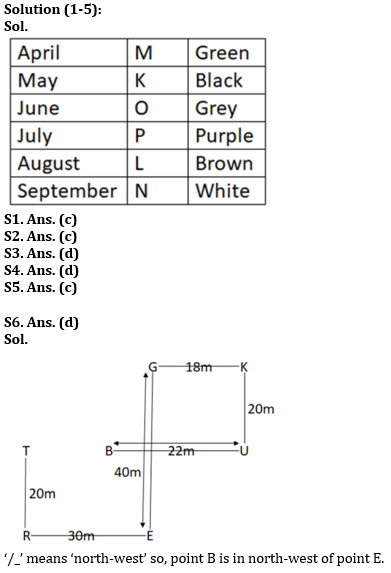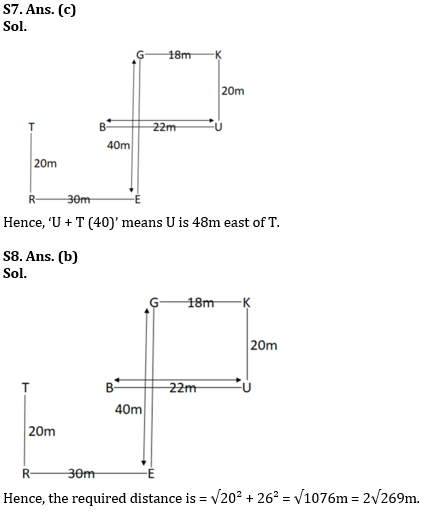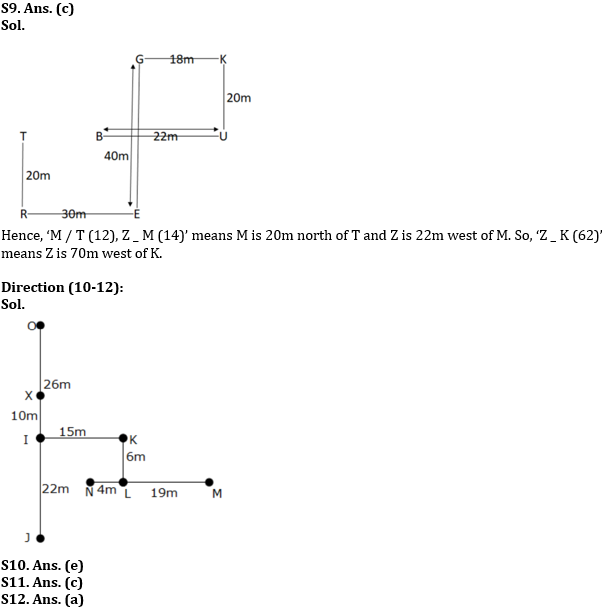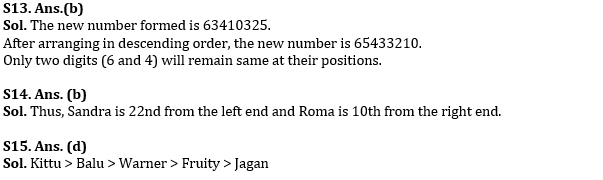#### Congratulations!Download Hindu Review of October 2021: Free PDF